# Energy of Electromagnetic Waves in Destructive Interference

• I
• MartinG
Gold Member
The most recent example is the alignment of the mirrors on the James Webb Space Telescope. In particular the final distance ("piston alignment") for each mirror segment had to be equal to within something like a tenth of a wavelength get the nice coherent center spot. They did okay I guess!
In this example - as for all optics - the intention is to get diffraction to give you enhancement of all light from one direction and cancellation from all other directions. Your 'main lobe' of directivity is pretty much the same for all reasonable reflectors (optical or radio) or lenses but the higher quality design will provide better and better cancellation of signals arriving off-axis (shaping and spreading 'side lobes' as much as possible to acceptable levels). It's a bit like an air mattress; you squash one part down and another part springs up but the volume of air inside stays the same.

•Delta2
keep_it_simple_silly
Hello Martin!

Many of the answers say that the conservation of energy is maintained because energy is 'redistributed' or goes to other location of constructive interference. This is an explanation which only roughly fits in certain cases but fails in some other.

The real explanation is not as straightforward as it seems. Although the energy of the whole system (source + receiver medium) is conserved, the energy radiated to the medium is not always the same or conserved!

In the case of YDSE or Young’s Double Slit Experiment, we observe that the average intensity of the fringes to be 2 times the Intensity of a single source. So far so good, then where’s the problem?

Now consider the following situations. Consider 2 sources are radiating waves of same frequency, same amplitude and no phase difference. :-

1. In case 1, the distance between these sources is λ/2, as shown in Figure 1.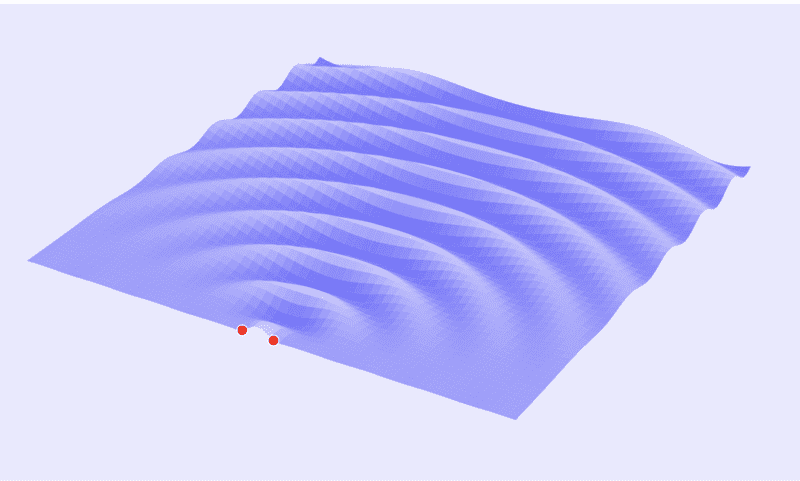2. then it is reduced to 0 in Figure 2.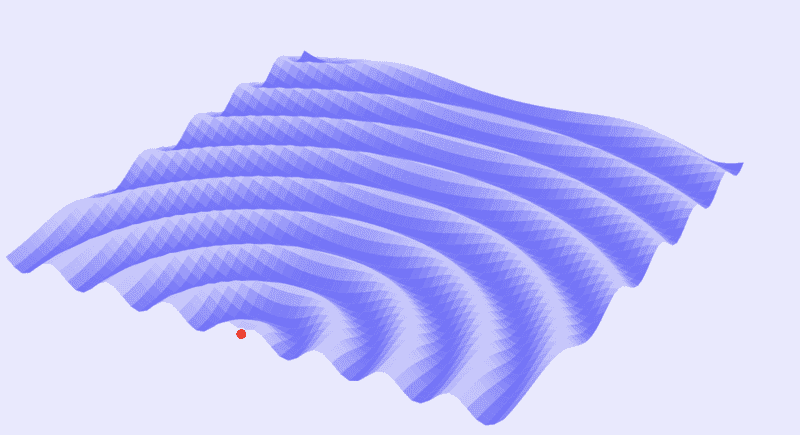3. If we calculate the power dissipated in these 2 cases, it turns out to be equal to 2*P in case of Figure 1, and 4*P in case of Figure 2. This clearly shows that by changing the distance, the net energy dissipated in medium is changing, not conserved.

We can qualitatively see this in Figure 1 and Figure 2. In Figure 1, The waves are absent in the sides, and in Figure 2, waves are present everywhere. This gives us the clear idea that more energy is being spent in case of Figure 2 The question is how?
• In order to give an explanation to this phenomenon, which fits all the cases and doesn't fail at any case, it is necessary to consider the ‘work done' by the source or the ‘wave impedance’. For example, do you think that a source generating a wave of amplitude A in a vacuum and a source generating the same wave in the environment when there is a varying electromagnetic field present at the source requires same amount of energy?
• This situation can be compared to pushing a rock on a plane surface vs pushing it on a hill. To get the same displacement, more energy needs to be transferred in case of pushing rock on the hill. This is because there is an ‘opposing force’ present in the latter case.
• In a similar way, when a source is generating a wave of amplitude 𝐴A when there is already a wave of amplitude 𝐴A present at the source, it needs to provide more energy to the system. The additional energy it is providing, in this case, is KA^2. So total energy provided by one source becomes 2KA^2, and when we add the energy from both sources, the net energy becomes 4KA^2.
• The case of 2 sources of opposite phases present at the same point, can be compared to pushing a rock down the hill. Because of the opposite nature of the field present at the source, the total work done by the source will become 0, and that is why there is no net radiation present, radiation being the transfer of energy to the medium by the source.
•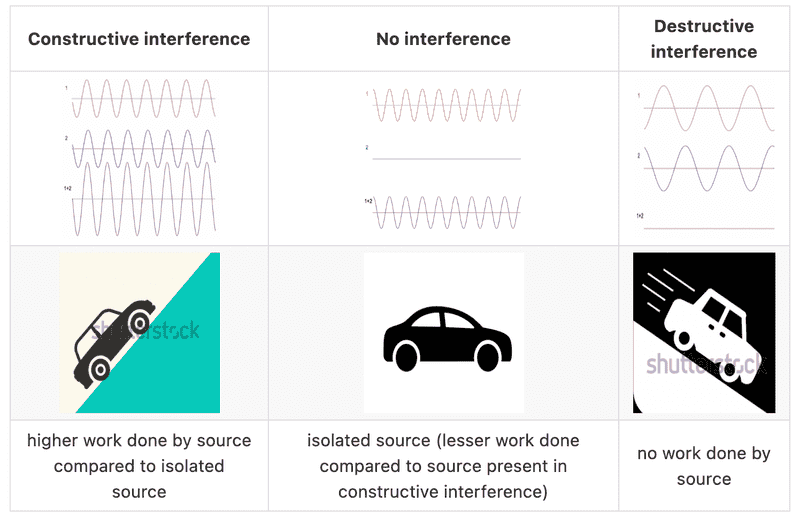• The concept of work done by the source was explained using term wave impedance by Levine in 1980 Ref, it’s explained in ref written by Authors Robert Drosd, Leonid Minkin, and Alexander S. Shapovalov

[Link to personal web blog redacted by the Mentors]

• Hope this helps! thank you!

Last edited by a moderator:
•weirdoguy
Gold Member
Now consider the following situations. Consider 2 sources are radiating waves of same frequency, same amplitude and no phase difference. :-
That is only possible if the two sources are in exactly the same place. There will always be regions where two real sources are in anti phase. So there's no need to worry that Physics got this wrong.

In that situation, the two sources have to be in phase or power will flow from one source into the other, which can make a lot of smoke at times, and accounts for your apparent energy imbalance

So I think your argument fails on the grounds that you haven't considered all contributions to your energy equation.

•Homework Helper
2022 Award
The real explanation is not as straightforward as it seems.
I have no idea what you are saying here but I fear it is largely not relevant. Suppose I have two identical point sources separated by ##\vec d##, and I consider the intensity at x where ##\vec x \cdot \vec d =0## i.e. perpendicular to ##\vec d##. If the sources are out of phase there will be destructive interference for all wavelengths (and at any value for d). It goes elsewhere.

•berkeman
Homework Helper
Gold Member
Many of the answers say that the conservation of energy is maintained because energy is 'redistributed' or goes to other location of constructive interference. This is an explanation which only roughly fits in certain cases but fails in some other.
I disagree with this statement. I think the "answers" that were given in the previous discussion are very accurate, and the mathematics clearly shows the energy gets redistributed in cases of interference where there is energy conservation.

•sophiecentaur, hutchphd and berkeman
Homework Helper
Gold Member
It might be worth mentioning that, when two sinusoidal point sources are used, (each with a given amplitude ## E_o ##, and each radiating spherically), there will be an increase in the radiated power of the system as the distance between them becomes smaller. If the separation is fairly large, then to a very good approximation the total radiated power stays the same regardless of the separation, and the interference pattern will result in a redistribution of the energy.

(If I have analyzed it correctly, there isn't a sudden discontinuity when the sources are superimposed, but rather an increase in power occurs as the separation decreases, and then the power nearly doubles when the separation is very small. [Edit: The relative phases of the sources is also going to play a role. I believe this one might get a little complicated, and I haven't analyzed it in its entirety.])

I agree with @sophiecentaur in post 38 that the energy needs to be considered very carefully. e.g. When the point sources get too close together, the power needs to be re-computed, and you can't simply assume that the power is what it was when the sources were further apart.

@keep_it_simple_silly may also have been trying to make a statement to that effect, but it wasn't totally clear on first reading.

Last edited:
keep_it_simple_silly
Hello Martin!

Many of the answers say that the conservation of energy is maintained because energy is 'redistributed' or goes to other location of constructive interference. This is an explanation which only roughly fits in certain cases but fails in some other.

The real explanation is not as straightforward as it seems. Although the energy of the whole system (source + receiver medium) is conserved, the energy radiated to the medium is not always the same or conserved!

In the case of YDSE or Young’s Double Slit Experiment, we observe that the average intensity of the fringes to be 2 times the Intensity of a single source. So far so good, then where’s the problem?

Now consider the following situations. Consider 2 sources are radiating waves of same frequency, same amplitude and no phase difference. :-

1. In case 1, the distance between these sources is λ/2, as shown in Figure 1.View attachment 315822
2. then it is reduced to 0 in Figure 2.
View attachment 315823
3. If we calculate the power dissipated in these 2 cases, it turns out to be equal to 2*P in case of Figure 1, and 4*P in case of Figure 2. This clearly shows that by changing the distance, the net energy dissipated in medium is changing, not conserved.

We can qualitatively see this in Figure 1 and Figure 2. In Figure 1, The waves are absent in the sides, and in Figure 2, waves are present everywhere. This gives us the clear idea that more energy is being spent in case of Figure 2 The question is how?
• In order to give an explanation to this phenomenon, which fits all the cases and doesn't fail at any case, it is necessary to consider the ‘work done' by the source or the ‘wave impedance’. For example, do you think that a source generating a wave of amplitude A in a vacuum and a source generating the same wave in the environment when there is a varying electromagnetic field present at the source requires same amount of energy?
• This situation can be compared to pushing a rock on a plane surface vs pushing it on a hill. To get the same displacement, more energy needs to be transferred in case of pushing rock on the hill. This is because there is an ‘opposing force’ present in the latter case.
• In a similar way, when a source is generating a wave of amplitude 𝐴A when there is already a wave of amplitude 𝐴A present at the source, it needs to provide more energy to the system. The additional energy it is providing, in this case, is KA^2. So total energy provided by one source becomes 2KA^2, and when we add the energy from both sources, the net energy becomes 4KA^2.
• The case of 2 sources of opposite phases present at the same point, can be compared to pushing a rock down the hill. Because of the opposite nature of the field present at the source, the total work done by the source will become 0, and that is why there is no net radiation present, radiation being the transfer of energy to the medium by the source.
• View attachment 315824
• The concept of work done by the source was explained using term wave impedance by Levine in 1980 Ref, it’s explained in ref written by Authors Robert Drosd, Leonid Minkin, and Alexander S. Shapovalov

[Link to personal web blog redacted by the Mentors]

• Hope this helps! thank you!
Follow the paper "Interference and the Law of Energy Conservation" by
Robert Drosd, Leonid Minkin, Alexander S. Shapovalov to know more

Gold Member
I disagree with this statement. I think the "answers" that were given in the previous discussion are very accurate, and the mathematics clearly shows the energy gets redistributed in cases of interference where there is energy conservation.
If you take a number of radio antennae and attempt to combine them with a given phase to produce a highly directional pattern by feeding them according to 'calculations', when the antennae are close together then the Mutual Impedance between them will, in the end, foil any attempt to make the array too directional. This is due to the effect that I mentioned higher up - they interact mutually and basically waste a lot of energy. You could say that that wasted energy has been directed out of the pattern.
It might be worth mentioning that, when two sinusoidal point sources are used, (each with a given amplitude Eo, and each radiating spherically), there will be an increase in the radiated power of the system as the distance between them becomes smaller.
Isn't that just because the local Impedance is not that of free space so Eo is not sufficient to work out the Energy flow. Actually, that seems to be an alternative to the Mutual Impedance I ,mentioned. Power will be dissipated within the sources. This is not an effect that kicks in at more than a half wave or so.

•keep_it_simple_silly
That is only possible if the two sources are in exactly the same place. There will always be regions where two real sources are in anti phase. So there's no need to worry that Physics got this wrong.

In that situation, the two sources have to be in phase or power will flow from one source into the other, which can make a lot of smoke at times, and accounts for your apparent energy imbalance

So I think your argument fails on the grounds that you haven't considered all contributions to your energy equation.
***That is only possible if the two sources are in exactly the same place. There will always be regions where two real sources are in anti phase. So there's no need to worry that Physics got this wrong.***

- not necessarily. Consider 2 sources in phase with a distance, say λ/8. In this condition, the combined energy dissipation is still greater than the sum of individual power dissipation for isolated sources. The formula which represents all cases is (formula is given by Vanderkooy and Lipshitz in their paper Power response of loudspeakers with noncoincident drivers – The influence of crossover design) :-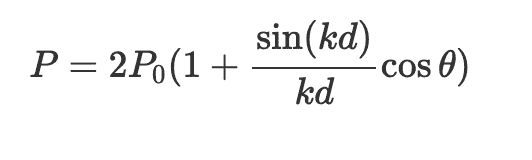Where,

1. P is total power radiated by both sources
2. P0 is the power radiated by single source in absence of other source
3. k=2π/λ, λ is wavelength of source
4. d is the distance between 2 sources
5. θ is the phase difference between 2 sources
If you plot graph for this particular equation, it looks like following for a phase difference of 0:-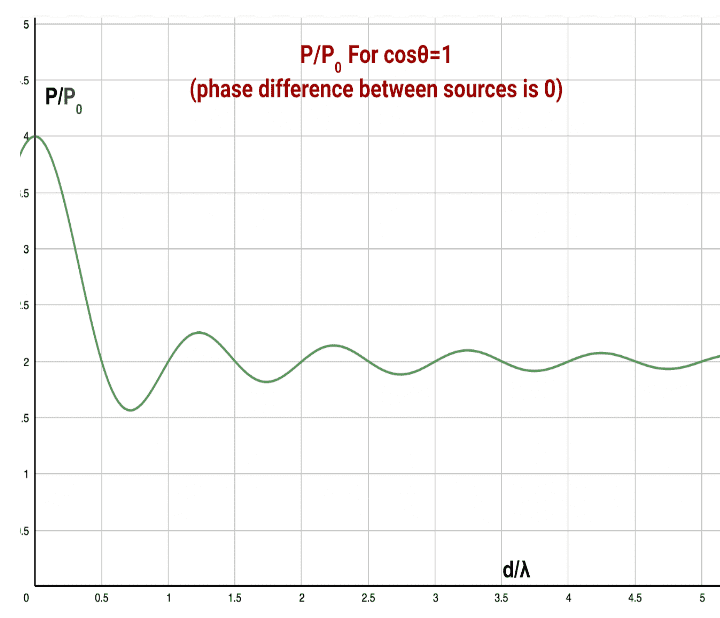So for any distance lesser than λ/2, power dissipation will be greater than 2P_0.
Check out this paper :-
Interference and the Law of Energy Conservation" by
Robert Drosd, Leonid Minkin, Alexander S. Shapovalov to know more
It makes things much clearer.

•Gold Member
2022 Award
•keep_it_simple_silly
keep_it_simple_silly
If you take a number of radio antennae and attempt to combine them with a given phase to produce a highly directional pattern by feeding them according to 'calculations', when the antennae are close together then the Mutual Impedance between them will, in the end, foil any attempt to make the array too directional. This is due to the effect that I mentioned higher up - they interact mutually and basically waste a lot of energy. You could say that that wasted energy has been directed out of the pattern.

Isn't that just because the local Impedance is not that of free space so Eo is not sufficient to work out the Energy flow. Actually, that seems to be an alternative to the Mutual Impedance I ,mentioned. Power will be dissipated within the sources. This is not an effect that kicks in at more than a half wave or so.

I disagree with this statement. I think the "answers" that were given in the previous discussion are very accurate, and the mathematics clearly shows the energy gets redistributed in cases of interference where there is energy conservation.
The 'redistribution of energy' explanation fits well when the distance between the sources, d>>λ but fails for cases when
d<<λ . Check out paper Interference and the Law of Energy Conservation as mentioned by @vanhees71.

•keep_it_simple_silly
It might be worth mentioning that, when two sinusoidal point sources are used, (each with a given amplitude ## E_o ##, and each radiating spherically), there will be an increase in the radiated power of the system as the distance between them becomes smaller. If the separation is fairly large, then to a very good approximation the total radiated power stays the same regardless of the separation, and the interference pattern will result in a redistribution of the energy.

(If I have analyzed it correctly, there isn't a sudden discontinuity when the sources are superimposed, but rather an increase in power occurs as the separation decreases, and then the power nearly doubles when the separation is very small. [Edit: The relative phases of the sources is also going to play a role. I believe this one might get a little complicated, and I haven't analyzed it in its entirety.])

I agree with @sophiecentaur in post 38 that the energy needs to be considered very carefully. e.g. When the point sources get too close together, the power needs to be re-computed, and you can't simply assume that the power is what it was when the sources were further apart.

@keep_it_simple_silly may also have been trying to make a statement to that effect, but it wasn't totally clear on first reading.
Yes, there is no sudden discontinuity in the power radiation as the distance between sources decreases.
The formula which represents all cases is (formula is given by Vanderkooy and Lipshitz in their paper Power response of loudspeakers with noncoincident drivers – The influence of crossover design) :-Where,
1. P is total power radiated by both sources
2. P0 is the power radiated by single source in absence of other source
3. k=2π/λ, λ is wavelength of source
4. d is the distance between 2 sources
5. θ is the phase difference between 2 sources
If you plot graph for this particular equation, it looks like following for a phase difference of 0:-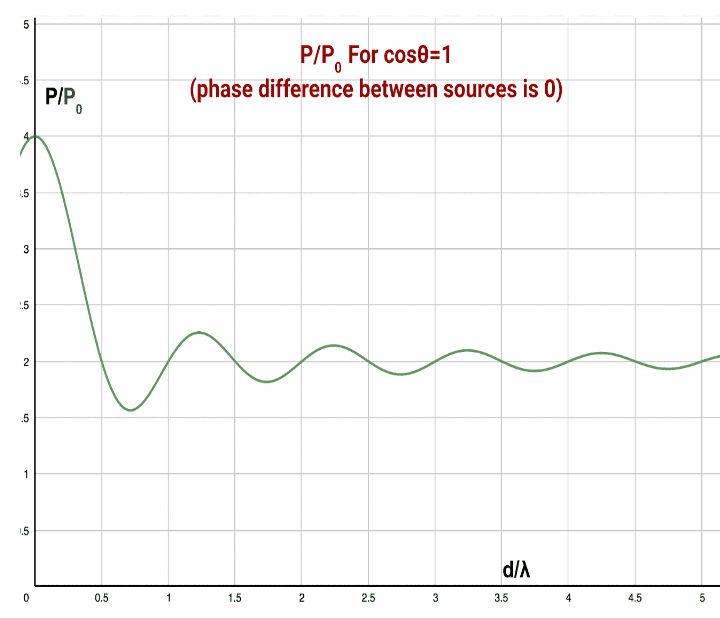you can see for larger distances(d>>λ), it is converging to 2P_0, and this is where the 'energy redistribution' argument roughly fits. Why rough? because you can see the graph still oscillating, but with minimum amplitude.
Im not sure if external links will be allowed by moderators but if they allow:- https://hackmd.io/@sharada10/wave_interference_energy_conservation

•Homework Helper
Gold Member
It may be worth mentioning that for the optics case, with a double slit experiment or with a diffraction grating, the sources are in the forward direction and the energy conservation and redistribution is a little simpler than with the r-f antenna. In any case, even with the optics case, we don't always get the simple and ideal case of energy conservation and redistribution. One case comes to mind that for very narrow slits the diffraction pattern can't expand any further (than ## \pi ## radians) as the slit narrows, (to compute the power which needs to be the same as the input power, the peak of the pattern is multiplied by the effective angular spread=the mathematics becomes problematic when the angular spread can't increase any further as the slit width narrows), and thereby the simple textbook diffraction theory for the case of very narrow slits is only a fairly good approximation.

Last edited:
•keep_it_simple_silly and vanhees71
Gold Member
The problem with this is that the Power in the wave depends on the impedance as well as the E field. P0 is only P/2 when the sources are far enough apart to ignore interactions. This expression:doesn't include the impedances involved - there's more to it than just the spacing. Both E and H fields count and also the source impedance, self Impedances of the antennae and also the mutual impedance. So that expression 'just' a system that may produce the right answers under some circumstances.

I'd be very suspicious of any work that suggests non-conservation of Energy. In the case of radiating systems, calculations are always of limited accuracy and they always require numerical solutions so I'd suggest that there will be a flaw that remains to be found.

•Homework Helper
Gold Member
Now that there has been a considerable addition and perhaps correction to what I said in post 4, I would like to also have you take another look at what I said in post 2, which I think is indeed correct, and where the optics texts often come up short in this interference matter:
In many textbooks, the Fabry-Perot effect is treated as an interference that is the result of the multiple reflections that occur on a slab of thickness ## d ##. Changing the thickness changes the phases of the multiple-reflected beams as they add to interfere with each other. Meanwhile, the Michelson interferometer is sometimes taught as the interference of the original source superimposed on its mirror image. Both of these are sort of ok, but they both can be presented in the same and more fundamental way as the result of the interference that occurs from two beams incident on a single interface from opposite directions. There is an interference here, and the energy redistribution will depend on the relative phases of the two beams.
[Edit: Note for each incident beam, there are exiting transmitted and reflected E-field sinusoidal components, (each with their own phase), that are computed using the Fresnel coefficients].
I attempted to present this more fundamental concept in the Insight that I authored a couple years ago (the link is in post 2), but perhaps you can follow my short explanation here of the interference that occurs that indeed conserves energy when two sinusoidal sources are incident on a single interface. The energy redistribution depends upon their relative phases.

With this "new" way of looking at it, the Fabry-Perot effect and Michelson interferometer, and a beamsplitter with two incident beams are all explained by the same fundamental concept.

[Edit: Note that many of the textbooks do present the topic with the Fresnel coefficients, e.g. with the Fabry-Perot effect, but they do not emphasize or show in detail what results if we simply consider the interference (i.e. energy redistribution) of the simplest case of two beams incident on a single interface from opposite directions.]

[Edit: and note that for this interference with a single interface, we use the Fresnel coefficients that still work when two beams are present, but we no longer can use the energy reflection coefficient ## R ## which works for a single beam incident on the interface. When two beams are present, the energy redistribution can be anything, (the energy no longer obeys linear principles even though the E-fields still obey linear principles), and is determined by the relative phases of the incident beams.]

Last edited:
•keep_it_simple_silly
keep_it_simple_silly
The problem with this is that the Power in the wave depends on the impedance as well as the E field. P0 is only P/2 when the sources are far enough apart to ignore interactions. This expression:
View attachment 315986
doesn't include the impedances involved - there's more to it than just the spacing. Both E and H fields count and also the source impedance, self Impedances of the antennae and also the mutual impedance. So that expression 'just' a system that may produce the right answers under some circumstances.

I'd be very suspicious of any work that suggests non-conservation of Energy. In the case of radiating systems, calculations are always of limited accuracy and they always require numerical solutions so I'd suggest that there will be a flaw that remains to be found.
1. There is absolutely no violation of the conservation of energy. The only difference is the consideration of work done which is usually ignored.
2. The expression doesn't contain the terms of wave impedances, but the impedance is already taken care of in the equation.
For example, a source will experience the highest impedance when it experiences a wave of the same amplitude, frequency and phase, which also means when the 2 sources are put at the same point. Now the power radiated in this case is 4P_0 by both sources, so this already includes the additional power dissipation by the source to overcome the resistance. You can see the same with destructive interference and other interferences in between.
3. This formula hasn't considered parameters like heating effects or others.

•Homework Helper
Gold Member
I'm very glad to see a somewhat thorough discussion of the energy/power interplay in the case of the two radiating sources as presented by @sophiecentaur and @keep_it_simple_silly . Meanwhile please see the "Edits" that I added to post 50 above.

Gold Member
3. This formula hasn't considered parameters like heating effects or others.
So your sources are approximations, which is fine, and they do the job that's required, apparently but you don't need to suggest departure from that basic conservation of energy principle to explain why the energy is apparently disappearing.

I always try to reach for the simplest wave systems available and RF antenna fill the bill well. They are pretty good omni radiators (in one plane) and they radiate coherent signals. Also, the Impedances can be calculated with some accuracy (a lot better than narrow slits, for instance). When some Power appears to be missing then the realities of non perfect transmitter amplifiers is somewhere to look. The same thing will apply to acoustic experiments with multiple speakers.

•Homework Helper
Gold Member
This thread is a couple months old, but I thought of another case that the OP and others might be interested in that involves interference and diffraction, and it also illustrates the conservation of energy that occurs in the formation of constructive and destructive interference: When a collimated beam (parallel rays) is focused to a small spot by a paraboidal mirror=[not a point as geometric (ray trace) optics would yield, but rather a diffraction limited spot], the energy is conserved as shown by calculations using diffraction theory for computing the intensity pattern in the focal plane. The (effective) area of the focused spot is finite, and is inversely proportional to the cross-sectional area of the beam/focussing optic. Meanwhile the intensity of the focused spot is proportional to the second power of the cross-sectional area of the beam/focusing optic, thereby conserving energy, as more detailed calculations show very precisely.
See https://www.physicsforums.com/insights/diffraction-limited-spot-size-perfect-focusing/

Edit: It should be noted that constructive interference occurs at and near the focal point of the paraboidal mirror because the optical path distance of every ray to the focal point is identical, (with each ray reflecting off the mirror with angle of incidence =angle of reflection).

Last edited:
•vanhees71 and hutchphd
Homework Helper
Gold Member
@MartinG , @sophiecentaur, @keep_it_simple_silly You might find the above post of interest. The energy conservation that occurs when light is brought to a focus by a lossless focusing optic (diffraction theory of optical imaging) is another example of the conservation of energy that occurs in optical interference as was also illustrated by post 44 above.

•vanhees71